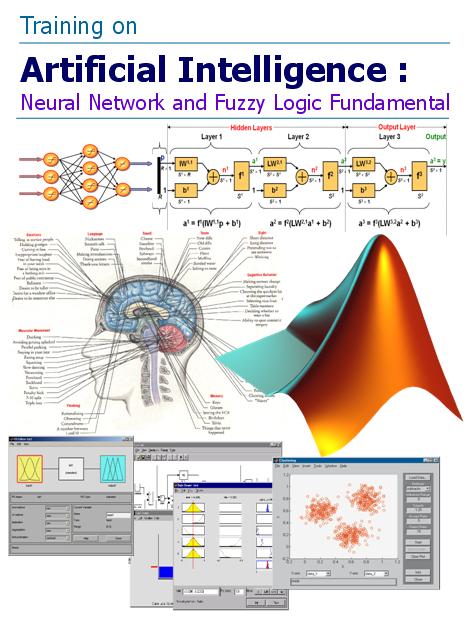#### Training onArtificial Intelligence Neural Network and Fuzzy Logic Fundamental Concepts and Techniques in MATLAB

Solutions 4U Training Center @ Puchong , Malaysia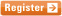13 & 14 JAN 2021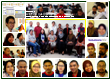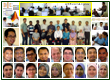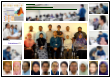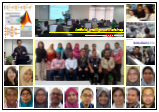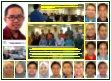" It is the science and engineering of making intelligent machines
especially intelligent computer program. It is related to the similar task of
using computers to understand human intelligence..."
 Artificial Intelligence (AI) may be regarded as an attempt to understand the processes of perception and reasoning that underlie successful problem solving and to incorporate the result of this research in effective computer programs. At present, AI is largely a collection of sophisticated programming technique that seek to develop systems that attempt to mimic human intelligence without claiming an understanding of the underlying processes involved. Artificial Intelligence (AI) can offer may advantages over traditional methods, such as statistical analysis, particularly where the data exhibits some form of non-linearity. Some existing application of spatial analysis and modeling techniques includes artificial neural networks and rule-based system fuzzy logic . Neural Network are biologically inspired and it is based on a loose analogy of the presumed working of a brain. However, if the working of the human brain are to be simulated using Neural Network, clearly, drastic simplifications must be adopted.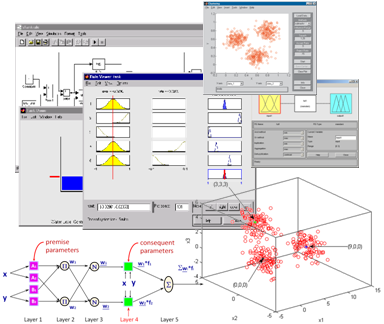The power of the human brain is a function of its ability to efficiently process imprecise information. To introduce this Fuzzy logic to a computational setting, the critical elements are not numbers but fuzzy sets. Which allow for quick processing of information by association of vaguely similar patterns while providing the mean to deal scientifically with subjectivity. This method has an advantage over Boolean logic in that it mimics complex human reasoning in order to arrive at realistic conclusions about the imprecise and often fuzzy nature of reality. Course Objectives “Artificial Intelligence: Neural Networks and Fuzzy Logic Fundamentals” is a two days workshop that focus on fundamental concepts and techniques for approaching artificial intelligence. This course will first discusses the fundamental principle of neural network and fuzzy logic, and then gives insight to tools available in MATLAB & SIMULINK to solve the complex and dynamic real-world problems. Numerous examples will be given to support the discussed theory.

Course Outline

Neural network concepts
Introduction to Neural Network
Simple neuron model
Representation of neural network in MATLAB

Types of learning methods
The Steepest Descent algorithm
The Back-propagation algorithm

Types of neural network
Perceptrons Architecture
Training of Perceptrons
Architecture of Linear Networks
The Widow-Hoff Learning Algorithms
Back-propagation Network Architecture
Training algorithms
Pre and Post processing
Competitive Learning
Self-Organizing Feature Map
Application examples

Case Study on Neural Network

Fuzzy logic concepts

Introduction to Fuzzy Logic
Fuzzy sets
Membership functions
Logical operations
If-Then rules

Fuzzy inference systems (FIS)
Introduction to FIS
Building FIS with Fuzzy GUI
Working from the command line
Application examples

Sugeno-Type Fuzzy inference
The ANFIS editor GUI
Working from the command line
Application examples

Case Study on Fuzzy Logic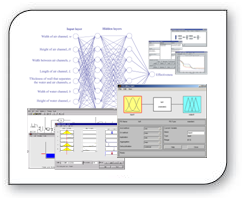Who Should Attend
Researchers, Lecturers, Scientists, Engineers and Managers that would want to use or plan to use artificial Intelligence, and to learn the fundamental knowledge in neural network and fuzzy logic using MATLAB.

Note: Due to the nature of the course, learning expectations, the availability seats are limited. You need to register early to obtain confirmation of your place.13 & 14 JAN 2021